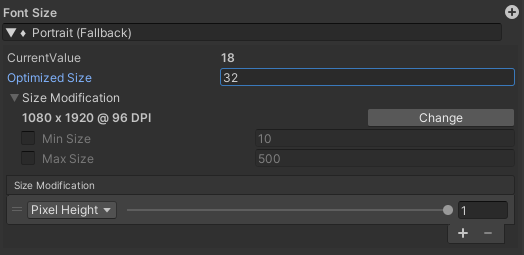# General

There are various "Sizer" objects on certain UI elements. They are used to scale certain values depending on the used resolution (and / or DPI).

Every "Sizer" contains the following values:

• Current Value: The last calculated value for the current resolution / dpi. Cannot be changed directly because it is calculated.
• Optimized Size: The fixed size for the optimized resolution / dpi
• Size Modification (Foldout)
• <X> x <Y> @ <Z> DPI [change]: The optimized resolution / dpi for this unity project.

by pressing the change button the Resolution Monitor object will be selected where the global Optimized resolution / dpi can be changed (see here).

• Min Size: The minimum size (if the calculation for a resolution / dpi would return a lower value, the Min Size is used instead)
since Better UI 2.5: This is optional, default is off.
• Max Size: The maximum size (if the calculation for a resolution / dpi would return a higher value, the Max Size is used instead)

since Better UI 2.5: This is optional, default is off.

• Size Modification (List): Contains Setting entries of how the size should be calculated. If the list is empty, the size will always be the optimized size.

If there is more than one entry, the middle is calculated.Note that all Size Modifiers are implemented as Size Config Collections.

# Size Modification Settings

Each Modification Setting consists of two values: Mode and Impact.

The Mode defines which Screen-values should be used to calculate the actual size (in the very most cases "Pixel Height" or "Pixel Width")

The Impact defines how much the modification settings affects the change of the size (doesn't affect at zero; affects totally at one).

Depending on the current screen resolution / dpi, the value is recalculated using the size modification settings.

Here are the different Modification Modes in detail.

## Pixel Height

If the height of the current resolution is smaller than the optimized resolutions height, the size will get smaller.

If the height of the current resolution is greater than the optimized resolutions height, the size will get bigger.

## Pixel Width

If the width of the current resolution is smaller than the optimized resolutions width, the size will get smaller.

If the width of the current resolution is greater than the optimized resolutions width, the size will get bigger.

## Aspect Ratio

The aspect ratio is calculated the following way: aspectRatio = width / height

If the aspect ratio of the current resolution is smaller than the optimized resolutions aspect ratio, the size will get smaller.

If the aspect ratio of the current resolution is greater than the optimized resolutions aspect ratio, the size will get bigger.

## Inverse Aspect Ratio

The inverse aspect ratio is calculated the following way: aspectRatio = height / width

If the inverse aspect ratio of the current resolution is smaller than the optimized resolutions inverse aspect ratio, the size will get smaller.

If the inverse aspect ratio of the current resolution is greater than the optimized resolutions inverse aspect ratio, the size will get bigger.

## Dpi

DPI means "Dots Per Inch" or in other words "how many pixels can fit next to each other within one inch on the current screen".

If you choose to scale in DPI mode, the physical size will always stay the same.

Example: If you have an object which displays a ruler and you adjust the correct size for the optimized resolution / dpi and you resize that object by using a sizer which modifies the size by dpi, the ruler will have the correct physical size on every device (if the device provides the correct dpi for its screen).

## Static Method 1-5

Introduced in Better UI 2.1

A custom method is used to calculate the size.

# Size Modifier Types

There are Size Modifiers for the following types:

• Float
• Vector2
• Vector3
• Margin (Wrapper for RectOffset – used for Padding of Layout Groups)

For each dimension of a type (x, y, z or left, right, top, bottom) there are modification lists. If you want to adjust the size uniformly make sure to set the same mode to all dimensions (e.g. set all to "Pixel Height").